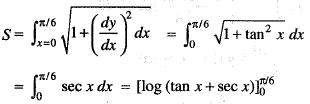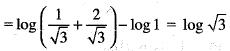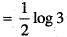Courses

# Integral Calculus -3

## 20 Questions MCQ Test Topic-wise Tests & Solved Examples for IIT JAM Mathematics | Integral Calculus -3

Description
This mock test of Integral Calculus -3 for IIT JAM helps you for every IIT JAM entrance exam. This contains 20 Multiple Choice Questions for IIT JAM Integral Calculus -3 (mcq) to study with solutions a complete question bank. The solved questions answers in this Integral Calculus -3 quiz give you a good mix of easy questions and tough questions. IIT JAM students definitely take this Integral Calculus -3 exercise for a better result in the exam. You can find other Integral Calculus -3 extra questions, long questions & short questions for IIT JAM on EduRev as well by searching above.
QUESTION: 1

### The integral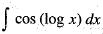equals:

Solution:

We have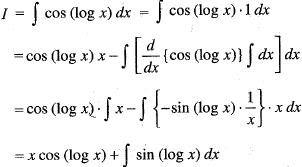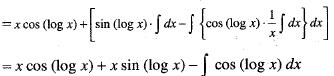So,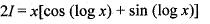implies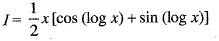QUESTION: 2

###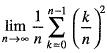is equal to:

Solution: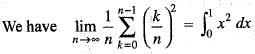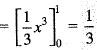QUESTION: 3

###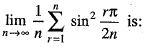Solution: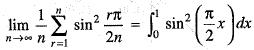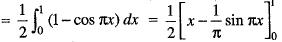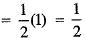QUESTION: 4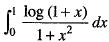is equal to:

Solution: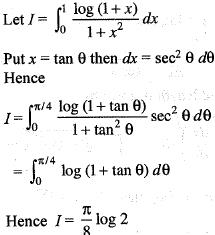QUESTION: 5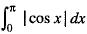is equal to:

Solution: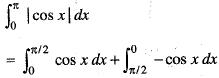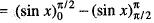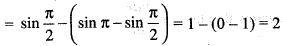QUESTION: 6

The value of definite integral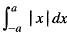is equal to:

Solution: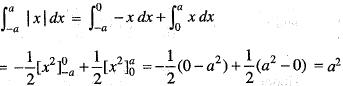QUESTION: 7

Let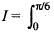sin2 ucos2 udu, then:

Solution: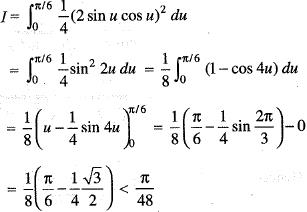QUESTION: 8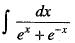is equal to:

Solution: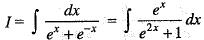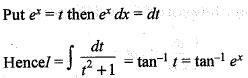QUESTION: 9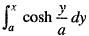is equal to:

Solution: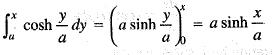QUESTION: 10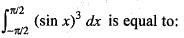Solution: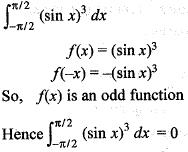QUESTION: 11

The moment of inertia of a hollow sphere about a diameter is:

Solution:

Moment of Inertia of a Hollow Sphere about the Diameter

Suppose the mass of a hollow sphere is M, ρ is the density, inner radius R2 and outer radius R1

∴ M = 4/3π(R1− R23

Moment of inertia of a hollow sphere (I) = Moment of inertia of a solid sphere of radius R1 - Moment of inertia of a solid sphere of radius R2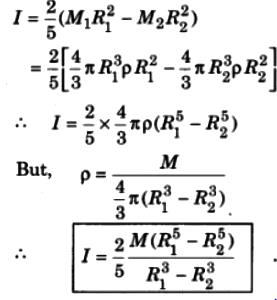QUESTION: 12

The area bounded by the curve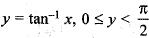from x = 0 to x = 1, the x -axis and the ordinate at x = 1 is:

Solution: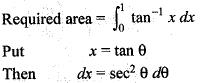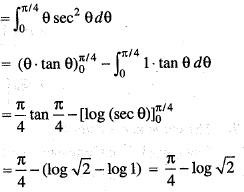QUESTION: 13

The area of the closed curve x = a cos t, y = b sint is given by:

Solution:

x = acost  ,  y = bsint

→  a2/x2​ + b2/y2​=1

It's a hyperbola whose area in any quadrant is given by πab/4​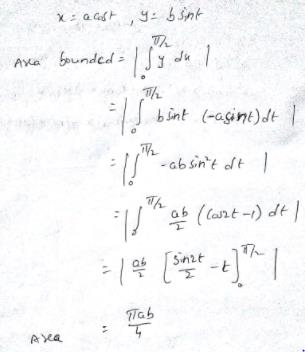QUESTION: 14

The area bounded by the curves y = ex, y = e-x and the line x = 1, is:

Solution:

Given curves are
y = ex
y = e-
From eqn (i) and (ii), we get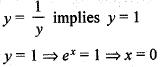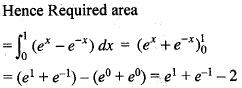QUESTION: 15

The intrinsic equation of the curve p = r sin α is given by:

Solution:

Given curve is p = r sin α   ....(i)
Hence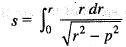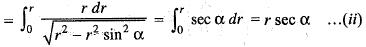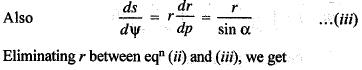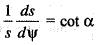or,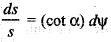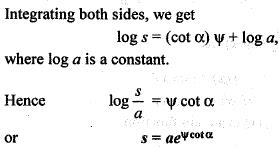QUESTION: 16

The area of surface of the solid generated by the revolution of the line segment y = 2x from x = 0 to x = 2, about x-axis is equal to:

Solution:

Required surface area is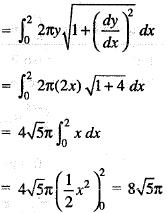QUESTION: 17

The perimeter of the curve r = 2 cos θ is:

Solution:

The curve is r = 2 cos θ
Changing it into Cartesian coordinate, we get
x2 + y2 = 2x
or, (x - l)2 + y2 = 1,
which is a circle with centre (1,0) and radius 1.
Hence
Perimeter = 2πr = 2π · 1 = 2π

QUESTION: 18

The length of the arc of the parabola x2 = 4ay from the vertex to the extremity of the latus rectum is given by:

Solution:

Given curve is x2 = 4ay
Differentiating, w.r. to y, we get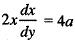implies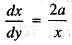implies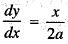Hence Length of the arc between vertex and extremity of latus rectum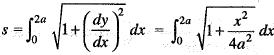QUESTION: 19

The area bounded by the curve y = x3, x -axis and the line x = 1 and x = 4 is given by:

Solution:

Given curve is y = x3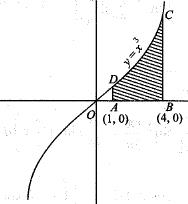The required area = Area ABCD =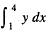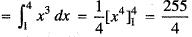QUESTION: 20

The length of the arc of the curve y = log sec x between x = 0 and x = π/6 is equal to:

Solution:

Given curve is y = log sec x
So,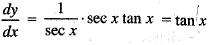Hence Required length is given by